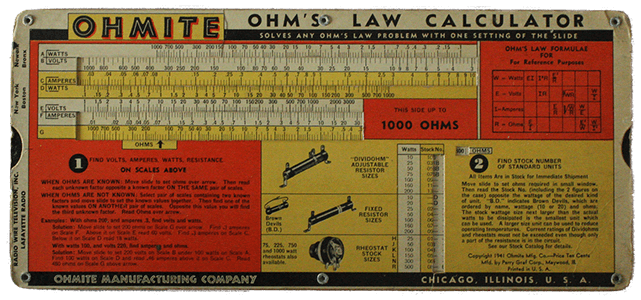Learning Objective

In this lesson we will learn about electrical resistance and how it can be calculated.

Learning Outcomes

By the end of this lesson you will be able to:

• Define electrical resistance and give examples of materials with high and low resistance.

• Describe how different factors can affect the resistance of an electrical wire.

• Describe fixed and variable resistors.

• Calculate total resistance in series and parallel circuits.

• Use Ohm’s law to calculate resistance, voltage and current.

## Contents

1 | Electrical Resistance

2 | Conductors and Insulators

3 | Factors Affecting Resistance of a Wire

4 | Resistors

5 | Calculating Total Resistance in a Circuit

6 | Ohm’s Law

7 | Summary(Image: bluebudgie, Pixabay)

PREVIEW THE WORKSHEET FOR THIS LESSON

PREVIEW THE YEAR 9 PHYSICS WORKBOOK